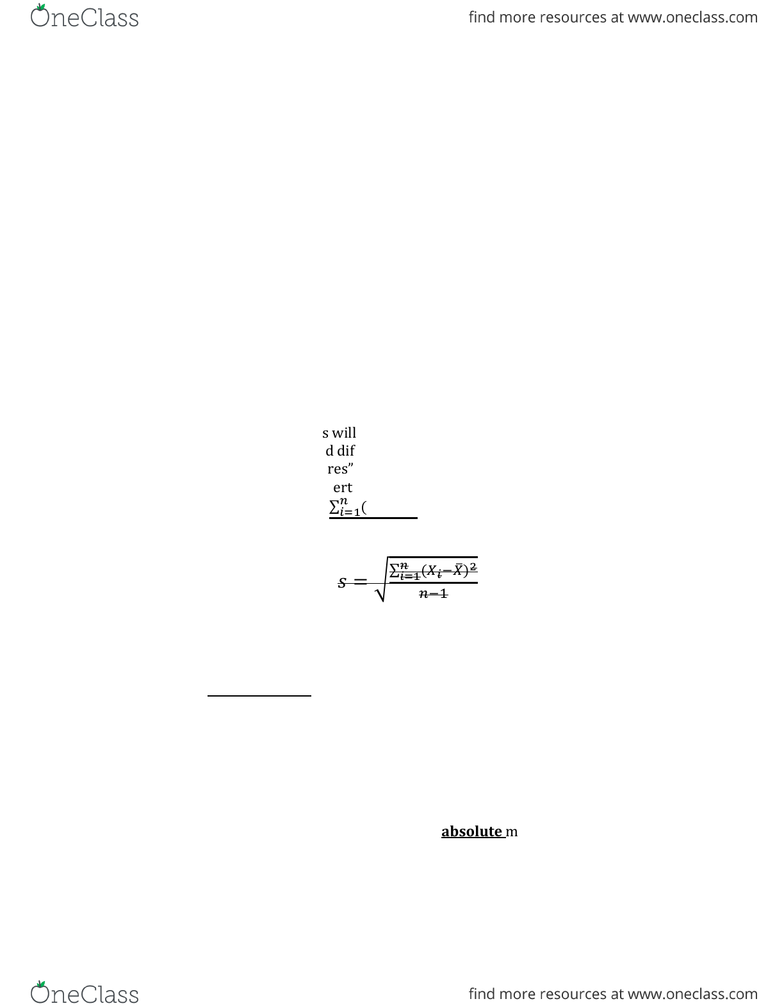# GGR270H1 Lecture Notes - Census Tract, Standard Deviation, Skewness

20 views3 pages
School
Course
Professor
Page:
of 3Measures of Dispersion
Range
Simplest measure of dispersion
Takes difference between smallest and largest value in the dataset, at the
interval/ratio scale
But, influenced by outliers
Range = Xmax - Xmin
Quartiles
Data are divided into quartiles (4 Groups)
Centiles (5 groups), Percentile (100 groups)
Standard Deviation and Variance
Two of the most commonly used measures of dispersion
Comparing values of each measure to the Mean
Xi X (x bar)
Two key properties of the mean/ value relationship
o Sum of differences will always add up to Zero
o Sum of the squared difference will be the minimum sum possible.
Called “Least Squares property
o Least Squares property carries over into calculating
o Variance: 
 , second X has a bar ontop , x bar =
sample mean
o Standard Deviation 

Skewness
o Measures the degree of symmetry in the frequency of distribution
o Determine how evenly (or unevenly) the values are distributed either
side of the mean
o 

o You can only say if something is positively skewed or negatively
skewed, there is no value of the difference
Coefficient of Variation
o Allows for comparison of variability spatial samples samples tied to
a particular location
o Tests which sample has the greatest variability
o Standard Deviation or Variance are absolute measures, so they are
influenced by the size of the values in the dataset can’t compare
among the samples because the scales will be different
o Used for when you want to compare 2 locations. Ex. studying
climatology and you are looking at rainfall in different areas, so you
want to find which location has the greatest variability in rainfall?? Or
looking at average household income, census data can be measured at
the census tract level. And look at the variability in the census tracts,
to see which one has the most variability
o To allow a comparison of variation across two or more geographic
samples, can use a relative measure of dispersion called Coefficient
of Variation
o CV = S/X (standard deviation/ mean)
o Example:
o
o Which station has the greatest degree of variation??
Station C because it has a greater CV
Practical Significance of Standard Deviation
Empirical Rule, lets say the grey area is
68% of the data, so it falls in one
standard deviation, so negative one
standard deviation and one standard
deviation
o 95% will fall in negative 2
standard deviation to 2 standard
deviation
o this is a universal law for the
distribution
EXAMPLE: Mean = 20 and S = 5
o So 68% will fall between 20 +/- 5
or between 15-25
o 95% fall between 10-30
o 99.7% fall between 5-35 (3
standard deviations)
o Normal Distribution Sample
PUT ON CHEAT SHEET
Z-Scores
Standard scores are referred to as Z scores
Indicated how many standard deviations separate a particular value from the
mean
Z scores can be + or depending if they are > or < the mean
Z score of the mean is 0 and the standard deviation is + or 1.
Table of Normal Values provides probability information on a standardized
scale.
o Statistics is a tool for forecasting, we can look at the probability of a
particular value. If we have the mean of the assignment and the
standard deviation, what is the probability of a student getting a
But we can also calculate Z scores
Formulas involves comparing values to the mean value, and dividing by the
standard deviation
Results is interoperated as the “number od standard deviations an
observation lies above or below the mean”
 
o Z is the standard score
o S is the standard deviation of the sample
o X each value in the data set
o X bar the man of all values in the data set
E.x. Rain fall in Toronto: the mean= 39.95 inches of rainfall , S =
7.5 inches
Z = 1.07. Therefore 48 inches is 1.07 standard
deviations above the mean. (If it were a negative value,
you would have said below the mean)